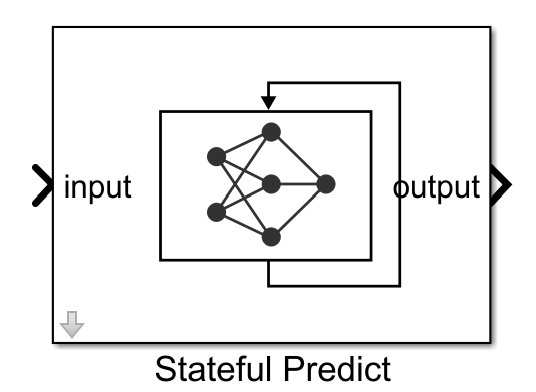# Stateful Predict

Predict responses using a trained recurrent neural network

Since R2021a

•Libraries:
Deep Learning Toolbox / Deep Neural Networks

## Description

The Stateful Predict block predicts responses for the data at the input by using the trained recurrent neural network specified through the block parameter. This block allows loading of a pretrained network into the Simulink® model from a MAT-file or from a MATLAB® function. This block updates the state of the network with every prediction.

To reset the state of recurrent neural network to its initial state, place the Stateful Predict block inside a Resettable Subsystem (Simulink) block and use the `Reset` control signal as trigger.

## Ports

### Input

expand all

The input ports of the Stateful Predict block takes the names of the input layers of the network loaded. Based on the network loaded, the input to the predict block can be sequence or time series data.

The dimensions of the numeric arrays containing the sequences depend on the type of data.

InputDescription
Vector sequencesc-by-s matrices, where c is the number of features of the sequences and s is the sequence length.
2-D image sequencesh-by-w-by-c-by-s arrays, where h, w, and c correspond to the height, width, and number of channels of the images, respectively, and s is the sequence length.

### Output

expand all

The outputs port of the Stateful Predict block takes the names of the output layers of the network loaded. Based on the network loaded, the output of the Stateful Predict block can represent predicted scores or responses.

For sequence-to-label classification, the output is a N-by-K matrix, where N is the number of observations, and K is the number of classes.

For sequence-to-sequence classification problems, the output is a K-by-S matrix of scores, where K is the number of classes, and S is the total number of time steps in the corresponding input sequence.

## Parameters

expand all

Specify the source for the trained recurrent neural network. The trained network must have at least one recurrent layer (for example, an LSTM network). Select one of the following:

#### Programmatic Use

 Block Parameter: `Network` Type: character vector, string Values: `'Network from MAT-file'` | `'Network from MATLAB function'` Default: `'Network from MAT-file'`

This parameter specifies the name of the MAT-file that contains the trained recurrent neural network to load. If the file is not on the MATLAB path, use the button to locate the file.

#### Dependencies

To enable this parameter, set the Network parameter to `Network from MAT-file`.

#### Programmatic Use

 Block Parameter: `NetworkFilePath` Type: character vector, string Values: MAT-file path or name Default: `'untitled.mat'`

This parameter specifies the name of the MATLAB function for the pretrained recurrent neural network.

#### Dependencies

To enable this parameter, set the Network parameter to `Network from MATLAB function`.

#### Programmatic Use

 Block Parameter: `NetworkFunction` Type: character vector, string Values: MATLAB function name Default: `'untitled'`

The Sample time parameter specifies when the block computes a new output value during simulation. For details, see Specify Sample Time (Simulink).

Specify the Sample time parameter as a scalar when you do not want the output to have a time offset. To add a time offset to the output, specify the Sample time parameter as a `1`-by-`2` vector where the first element is the sampling period and the second element is the offset.

By default, the Sample time parameter value is `-1` to inherit the value.

#### Programmatic Use

 Block Parameter: `SampleTime` Type: character vector Values: scalar | vector Default: `'-1'`

## Version History

Introduced in R2021a